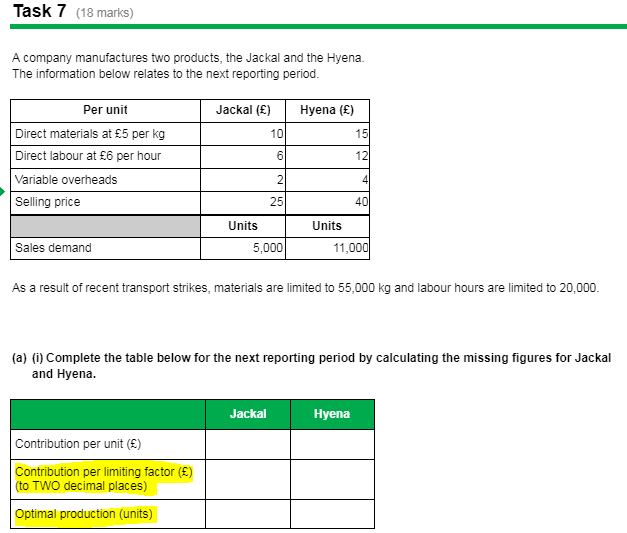AAT Assessment 1 Task 1.7a

Hi,

Please can someone help with how to get the answers for the parts I've highlighted.

Sophie• We need to establish what the limiting factor is first

Materials are 55000 kg and labour is 20000h

The Jackal takes 1 h to produce (6/6=1)
5000 units x 1h=5000h

Hyena take 2h (12/6=2), therefore 10000x2=20000h

The total hours required is 25000h

This is the limiting factor, so the contribution per limited factor is

For J 7/1h=7
Fir H 9/2h=4.5

Optimal units that can be produced will be

All of J as it has the highest contribution per limited factor, so 5000 units and whatever is left for H

5000 will take 5000h, so we have left 15000h/2h =7500 units of Hyena

AAT Level 2. 2018 Distinction
AAT Level 3. 2018 Distinction
AAT Level 4. 2019 FSLC 100% MABU 93% MADC 82% PDSY 80%
• dumutroba said:

We need to establish what the limiting factor is first

Materials are 55000 kg and labour is 20000h

The Jackal takes 1 h to produce (6/6=1)
5000 units x 1h=5000h

Hyena take 2h (12/6=2), therefore 10000x2=20000h

The total hours required is 25000h

This is the limiting factor, so the contribution per limited factor is

For J 7/1h=7
Fir H 9/2h=4.5

Optimal units that can be produced will be

All of J as it has the highest contribution per limited factor, so 5000 units and whatever is left for H

5000 will take 5000h, so we have left 15000h/2h =7500 units of Hyena

@dumutroba quick question why is it 10000 x 2 when Hyena sales demand is 11000?
• Misspelled. Is 11000x2h
AAT Level 2. 2018 Distinction
AAT Level 3. 2018 Distinction
AAT Level 4. 2019 FSLC 100% MABU 93% MADC 82% PDSY 80%